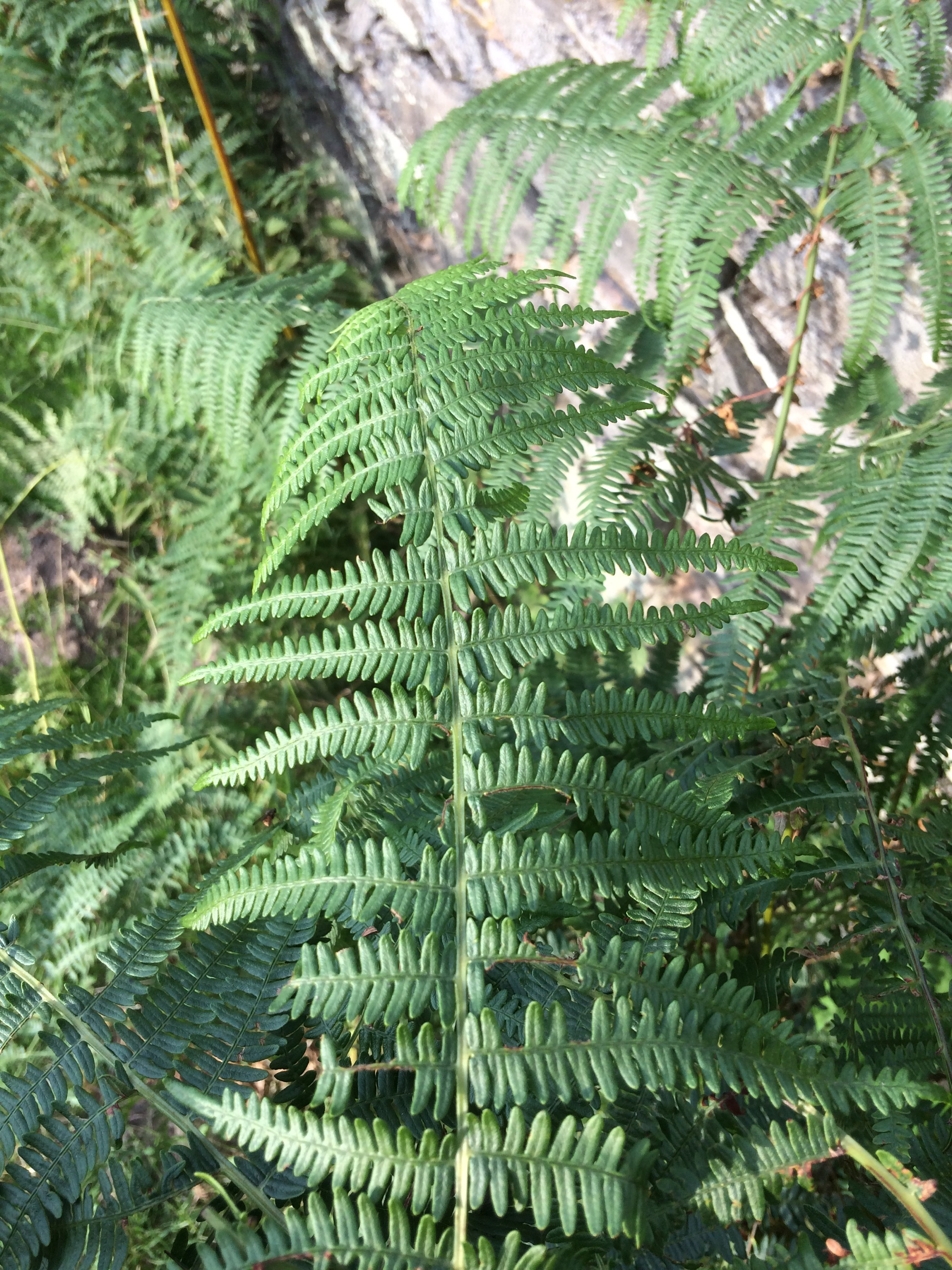Categories

# A Bit More on Barnsley’s Fern

After writing my original Matlab program to plot Barnsley’s fern I thought I would look into it in a bit more detail and read his original paper here and have had a play around with the parameters in the program. I have been trying to generate a fern that looks a bit like one that I saw at Bradgate Park in Leicestershire.After a while of adjusting the parameters and getting ferns that I wasn’t completely happy with I decided to look online and see if anyone had come up with some parameter sets already. It turns out they had, and using the given parameters for the Cyclosorus fern  I can come up with something pretty good.

In the notation of the previous post, the affine transformations are given by

\begin{align} f_1(x,y) &= \begin{pmatrix} 0.000 & 0.000 \\ 0.000 & 0.250 \end{pmatrix} \begin{pmatrix} x \\ y \end{pmatrix} + \begin{pmatrix} 0.000 \\ -0.400 \end{pmatrix} \\ f_2(x,y) &= \begin{pmatrix} 0.950 & 0.005 \\ -0.005 & 0.930 \end{pmatrix} \begin{pmatrix} x \\ y \end{pmatrix} + \begin{pmatrix} -0.002 \\ 0.500 \end{pmatrix} \\ f_3(x,y) &= \begin{pmatrix} 0.035 & -0.200 \\ 0.160 & 0.040 \end{pmatrix} \begin{pmatrix} x \\ y \end{pmatrix} + \begin{pmatrix} -0.090 \\ 0.020 \end{pmatrix} \\ f_4(x,y) &= \begin{pmatrix} -0.040 & 0.200 \\ 0.160 & 0.040 \end{pmatrix} \begin{pmatrix} x \\ y \end{pmatrix} + \begin{pmatrix} 0.083 \\ 0.12 \end{pmatrix} \end{align}

with associated probabilities $$0.02, 0.84, 0.07, 0.07$$. These give the following plot after a million iterations.Have play with the parameters and see what you can come up with. I have also found a very nice bit of html5 and javascript code that generates Barnsley’s ferns, this is here – take a look at it.